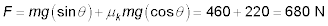##### Physics I Workbook For Dummies with Online Practice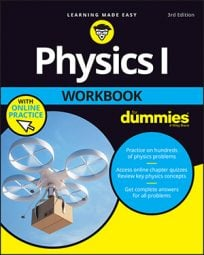Two kinds of friction — static and kinetic — mean that you have to handle ramp problems where kinetic friction is involved as well as problems where static friction is involved. Kinetic friction is involved any time an object is moving up or down a ramp.. You can solve problems with kinetic friction as easily as those that involve static friction.

Here’s the equation for the force needed to get an object moving and thus overcome static friction: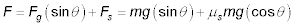To convert this equation to using kinetic friction, all you have to do is to change from using the coefficient of static friction to using the coefficient of kinetic friction: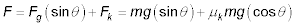That’s all there is to it.

## Sample question

1. Suppose that you’re pushing a 40.0-kg plastic crate up a 19.0-degree ramp.

The coefficient of kinetic friction is 0.100, and you need to apply a force to keep the crate moving. What is the force you will need to apply?

The correct answer is 165 N.

1. The equation here is2. Putting in the numbers gives you F =128 + 37 = 165 N.

## Practice questions

1. You’re dragging your little sister up the 25-degree wheelchair ramp at the doctor’s office.

If she has a mass of 30.0 kg and the coefficient of kinetic friction is 0.10, how much force will you need to apply to keep her going?

2. You’re pushing a box of books with a mass of 25 kg up a 40-degree ramp.

If the coefficient of kinetic friction is 0.27, how much force will you need to apply to keep the box moving up the ramp?

3. You’re pushing a chest of drawers up an 18-degree ramp.

If it has a mass of 50.0 kg and the coefficient of kinetic friction is 0.20, how much force will you need to keep it moving?

4. You want to keep a 120-kg refrigerator moving up a 23-degree ramp.

If the coefficient of kinetic friction is 0.20, how much force will you need to keep it moving?

Following are answers to the practice questions:

1. 150 N

1. Determine the forces you need to overcome: The force due to gravity is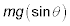and the force due to kinetic friction is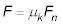You need to find the normal force.

2. The equation for normal force is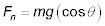Use the normal force to calculate the force due to kinetic friction: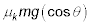3. The force you have to overcome is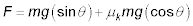4. Plug in the numbers: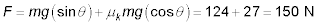2. 210 N

1. Calculate the forces you need to overcome: The force due to gravity isand the force due to kinetic friction isYou need to find the normal force.

2. The equation for normal force isUse the normal force to calculate the force due to kinetic friction:3. The force you have to overcome to keep the box moving is4. Plug in the numbers: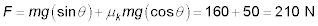3. 240 N

1. Calculate the forces you need to overcome: The force due to gravity isand the force due to kinetic friction isYou need to find the normal force.

2. The equation for normal force isUse the normal force to calculate the force due to kinetic friction:3. The force you have to overcome to keep the chest of drawers moving is4. Plug in the numbers: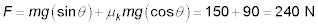4. 680 N

1. Calculate the forces you need to overcome: The force due to gravity isand the force due to kinetic friction isYou need to find the normal force.

2. The equation for normal force isUse the normal force to calculate the force due to kinetic friction:3. The force you have to overcome to keep the refrigerator moving is4. Plug in the numbers: